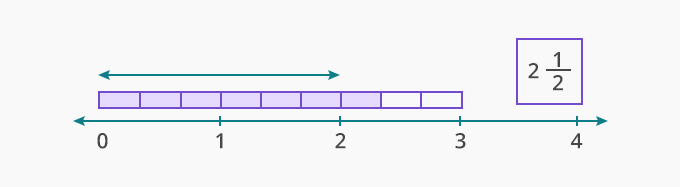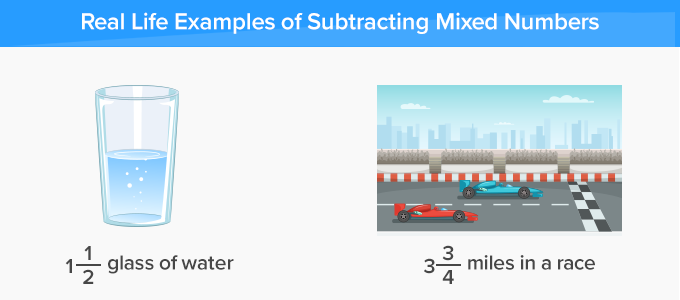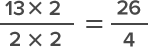Subtracting Mixed Numbers - Definition with Examples

The Complete K-5 Math Learning Program Built for Your Child

• 30 Million Kids

Loved by kids and parent worldwide

• 50,000 Schools

Trusted by teachers across schools

• Comprehensive Curriculum

Aligned to Common Core

Subtracting Mixed Numbers

Fractions which are more than a whole are known as mixed fractions or mixed numbers.

For instance: Nicholas has 3 chocolates, and each chocolate has 3 bars. He eats 7 bars of chocolate, which means 2 whole chocolates and 1 bar of the third chocolate.Real life examples of Subtracting mixed numbers:How to Subtracting mixed numbers?

The operation of subtracting mixed numbers is same as adding mixed numbers.

Case 1: Subtracting mixed numbers with same denominators

Follow the given steps to subtract mixed numbers with same denominators:

Step 1- Subtract the wholes.

Step 2- Convert the fractions into improper fractions.

Step 3- Subtract the fraction.

Step 4- Change the improper fraction into a mixed number if needed.

Step 5- Write the mixed number with wholes and the fraction.

Example 1: 5 73 - 3 23

Step 1 - Subtract 3 from 5.

5 - 3 =2

Step 2 - Subtract the fractions:

73 - 23

Step 3 - Write the fraction with whole.

5 73 - 3 2= 2 53

Case 2: Subtracting mixed numbers with different denominators

Follow the given steps to subtract mixed numbers with different denominators:

Step 1- Convert the mixed numbers into improper fractions.

Step 2- Find the common multiple of both the denominators.

Step 3- Convert the fractions as common denominators.

Step 4- Solve the fractions.

Step 5- Convert the fraction as a mixed number.

Example 2: 6 12 - 1 34

Step 1- Convert the mixed numbers into improper fractions.

1 32 - 74

Step 2- Find the common multiple of both the denominators 2 and 4.

Common multiple of 2 and 4 is 4.

Step 3- Convert the fractions as common denominators.Step 4- Solve the fractions

264 - 74 = 194

Step 5- Convert the fraction as a mixed number.

194 = 4 34

 Fun Facts We can multiply a fraction by any number, the value of the fraction always remains the same.

Won Numerous Awards & Honors